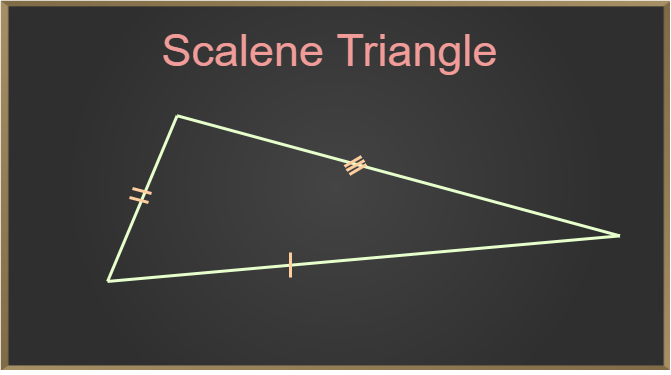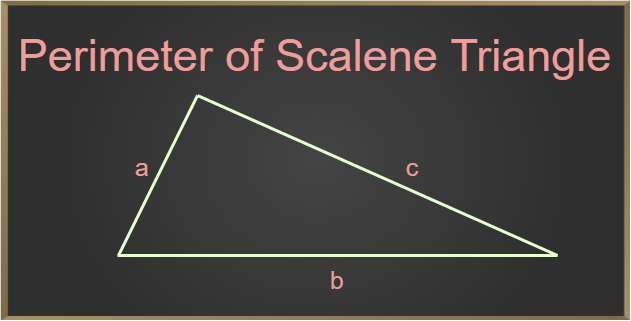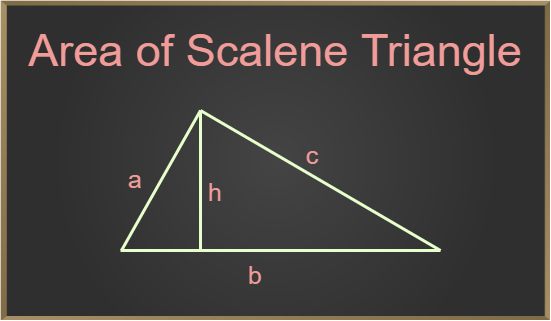GFG App
Open AppBrowser
Continue

A scalene triangle is one in which each of the three sides has a distinct length and each of the three angles has a different measurement. The total of all the internal angles of the scalene triangle, however, is unaffected by the various measurements. The sum of a scalene triangle’s three internal angles is always 180°, satisfying the triangle’s angle sum condition.

## What is a Scalene Triangle?

A scalene triangle is a triangle in which no two sides of a triangle are equal also, any of the two angles are not equal to each other in a scalene triangle. Interior angles of a scalene triangle follow the triangle sum property i.e. the sum of all the angles of a scalene triangle is equal to 180 degrees.## Scalene Triangle Formula

A triangle with no two sides equal is called a scalene triangle. A scalene triangle has two major formulas Perimeter of Scalene Triangle, Area of Scalene Triangle these two formulas are discussed below

## Perimeter of a Scalene Triangle

Perimeter of any figure is the length of its total boundary. So, the perimeter of a scalene triangle is defined as the sum of all of its three sides.From the above figure,

Perimeter = (a + b + c) units

where
a, b and c are the sides of the triangle.

## Area of a Scalene Triangle

Area of any figure is the space enclosed inside its boundaries for the scalene triangle area is defined as the total square unit of space occupied by the Scalene triangle. Area of the scalene triangle depends upon its base and height of it.A = (1/2) × b × h sq. units

where,
b is the base and h is the height (altitude) of the triangle.

If the lengths of all three sides of the scalene triangle are given instead of base and height, we calculate the area using Heron’s formula, which is given by,

A = √(s(s – a)(s – b)(s – c)) sq. units

where,
s denotes the semi-perimeter of the triangle, i.e, s = (a + b + c)/2,
a, b, and c denotes the sides of the triangle.

## Properties of Scalene Triangle

A scalene triangle is a triangle with all three sides of different lengths. The Sum of all three angles of the scalene triangle is 180 degrees. A few of the important properties of a scalene triangle are,

• All three sides of a scalene triangle are not equal.
• No angle of the Scalene triangle is equal to one another.
• Interior angles of a scalene triangle can be either acute, obtuse, or right angle, but some of all its angle is 180 degrees.
• No line of Symmetry exists in the Scalene triangle

## Difference between Equilateral, Isosceles, and Scalene Triangles

On the basis of sides, triangles are classified into three types –

• Equilateral Triangle
• Isosceles Triangle
• Scalene Triangle

The main differences between, these three types of triangles are,

## Solved Examples on Scalene Triangle

Example 1: Find the perimeter of a scalene triangle with side lengths of 10 cm, 15 cm, and 6 cm.

Solution:

We have, a = 10, b = 15 and c = 6.

Use the formula P = (a + b + c) to find the perimeter.

P = (10 + 15 + 6)

= 31 cm

Example 2: Find the length of the third side of a scalene triangle with two side lengths of 3 cm and 7 cm and a perimeter of 20 cm.

Solution:

We have, a = 3, b = 7 and P = 20.

Use the formula P = (a + b + c) to find the unknown length.

P = (a + b + c)

20 = (3 + 7 + c)

20 = 10 + c

c = 10 cm

Example 3: Find the area of a scalene triangle with side lengths of 8 cm, 6 cm, and 10 cm.

Solution:

We have, a = 8, b = 6 and c = 10.

Use the formula s = (a + b + c)/2 to find the semi-perimeter.

s = (8 + 6 + 10)/2

= 24/2

= 12 cm

Use the formula Area = √(s(s – a)(s – b)(s – c))

A = √(12(12 – 8)(12 – 6)(12 – 10))

= √(12(4)(6)(2))

= √576

= 24 sq. cm

Example 4: Find the area of a scalene triangle whose base is 20 cm and altitude is 10 cm.

Solution:

We have, b = 20 and h = 10

Area of a scalene triangle given its base and height Area = 1/2 × b × h

A = 1/2 × 20 × 10

= 100 sq. cm

Thus, the area of the given scalene triangle is 100 sq. cm.

## FAQs on Scalene triangle

Question 1: What is a scalene triangle?

Scalene triangles are triangles with all three sides unequal, i.e. in a scalene triangle, no two sides are equal.

Question 2: Can a scalene triangle be an obtuse-angled triangle?

Yes, a scalene triangle can be an obtuse-angled triangle. For an obtuse-angled triangle, any one angle is greater than 90° and the other two angles are less than 90° such that the total sum is 180° which is possible in a scalene triangle.

Question 3: What are the unique properties of the scalene triangle?

Properties of Scalene Triangle are:

• In a scalene triangle, all sides are unequal.
• A scalene triangle has no line of symmetry.
• For a scalene triangle, interior angles can be acute, obtuse, or right-angle.

Question 4: Write the formula for the area and perimeter of the scalene triangle.

Required formulas are:

Area of the scalene triangle = 1/2 × b × h
Perimeter of the scalene triangle = a + b + h

where,
a, b, c are its sides
b is the base and h is the height

Question 5: Does the angle-sum property holds true in a scalene triangle?